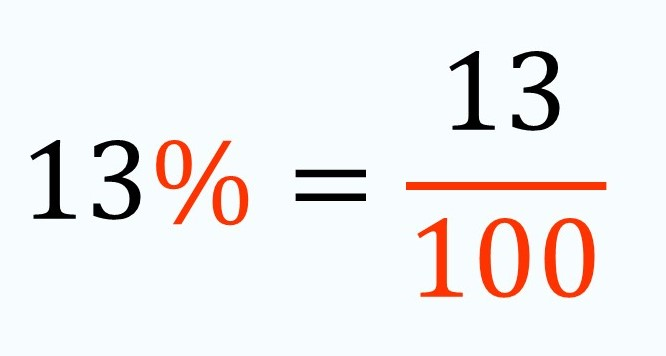Smartick is an advanced online program that teaches kids math and coding in only 15 min. a day

Oct15

# Percentage: What Is It and How do We Calculate It?

If we talk about percentages, it seems to be a familiar topic but: Would we know what a percentage is? How it is calculated? And what it means exactly? This post is going to resolve all these doubts, let’s get to it!

#### A percentage is, actually, a symbol.

A symbol that represents a fraction with 100 as its denominator. So, in written language, it is much easier to write the percentage rather than the fraction:We read this symbol (%) as ”percent” and it indicates, like we had said before, the number of parts of a unit, or a reference amount, that has been divided. Therefore, percentage (%) always appears in an expression that relates two quantities. For example, ”13% of children that do Smartick chose to play the game Entangled after their session.” In this case, the quantities that are being related are the number of children that do Smartick and the amount of those children that chose to play Entangled after their session.But, how could we calculate the exact number of children that chose to play Entangled? Well, it´s very simple, but we should know a few things:

• How to multiply fractions.
• How many children do Smartick: imagine that there are only 300 children.